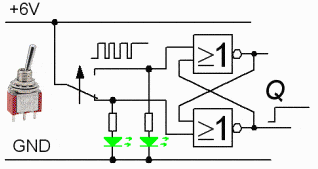# Logic Gates and Switches

Hello,

I'm currently studying a course in digital logic. The course is included in my IT program. And I have not taken a course in electronics yet; I will later.

My question basically is: what is the output of a logic gate given that one input is neither connected to a high voltage nor connected to ground? (That is, the input is not connected to anything.) And why?Tomorrow I'm having a lab, where I will connect a mechanical switch to a latch.

In general case the output will be set at high state or at low state or somewhere between these two states. It all be depend on the logic family you are actually be using. And this is why we should never leave unused inputs floating. And always use a pull-up or pull-down resistors.

Baluncore
The power supplies to the logic gates are implicit and so not usually shown. In this case the supplies are assumed to be GND = 0V and +6V. Since four gates, each with two inputs and one output, are all in each package, they can share the two power supply pins. That makes 3*4 + 2 = 14 pins on the IC.

The “>=1” gate is an OR gate, but it has an inverted output, so it is a NOR gate.
The circuit shown is an RS flipflop. If you follow the logic state through the circuit, then flip the switch and watch the new state develop, you will understand why this circuit is called a switch de-bounce circuit.

berkeman
Mentor
My question basically is: what is the output of a logic gate given that one input is neither connected to a high voltage nor connected to ground? (That is, the input is not connected to anything.) And why

It is generally considered a design error to leave logic inputs floating. Especially with CMOS gates, floating inputs can cause problems with increased power consumption and noise generation. Always tie off unused logic inputs.Baluncore